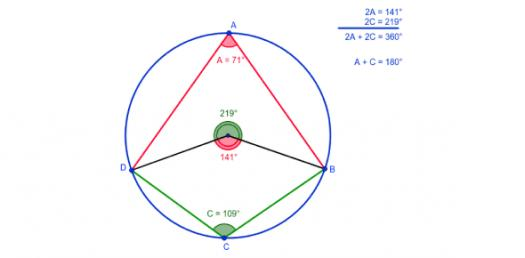# Circle Theorems Trivia Quiz: Can You Answer These Questions?

23 Questions | Total Attempts: 25Settings.

Related Topics
• 1.
x = [Blank]o
• 2.
y = [Blank]o
• 3.
x = [Blank]o
• 4.
y = [Blank]o
• 5.
x = [Blank]o
• 6.
y = [Blank]o z = [Blank]o
• 7.
x = [Blank]o y = [Blank]o
• 8.
z = [Blank]o
• 9.
x = [Blank]o
• 10.
y = [Blank]o
• 11.
x = [Blank]o
• 12.
y = [Blank]o
• 13.
x = [Blank]o
• 14.
y = [Blank]o
• 15.
x= [Blank]o
• 16.
y = [Blank]o z = [Blank]o
• 17.
x = [Blank]o y = [Blank]o
• 18.
z = [Blank]o
• 19.
x = [Blank]o
• 20.
y = [Blank]o
• 21.
x = [Blank]o y = [Blank]o z = [Blank]o
• 22.
x = [Blank]o
• 23.
y = [Blank]o